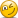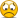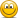# Fresh quiz of Tuckman's (Chapter 7) Term Structure Case

#### David Harper CFA FRM

##### David Harper CFA FRM
Staff member
Subscriber
In the discussion associated with our T5-40 question at old https://forum.bionicturtle.com/threads/l2-t5-40-replicating-callable-bond-tuckman.3551/post-78646 @Sixcarbs asked for a fresh situation (same mechanics but simply with different but coherent numbers). Here is what I've come up with

Assume the following:
• Currently the six-month spot rate, S(0.5) = 3.00%, and one-year spot rate, S(1.0) = 3.10%
• Our presumed binomial interest rate tree characterizes (short-term) six-month rates and has only two steps, so each step is also six-months and the tree only extends out to one year. At each node, six-month rate jumps up or down 40 basis points with equal probability. The initial six-month rate is 3.00%. In six months, then, the new six-month rate will either be 3.40% (with 50% probability) or 2.60% (with 50% probability).
• We are evaluating a one-year zero-coupon bond with a face value of $1,000 and a call option on this bond with a strike price of$985.00; the option matures in six months (like Tuckman's example).
• I believe my mechanics match Tuckman's Chapter 7 example, but of course my values are different. For comparison,
• His spot rates are S(0.5) = 5.00% and S(1.0) = 5.15%
• His six-month binomial rate tree starts at 5.00% and jump up/down by 50 bps with equal probability; i.e., to 5.50% or 4.50%
• His bond has a face value of $1,000.00 and his six-month option has a strike price of$975.00
Questions:
1. What is the bond's (theoretical) market price?
2. We are told (given) that the real-world (aka, true) probabilities are 50%/50%, but what are the risk-neutral probabilities?
3. If we use the real-world probabilities, what is the expected discounted value of the call option?
4. If we use the risk-neutral probabilities, what is the market price of the call option?
5. How can we interpret the risk-neutral probabilities?
=================================
Solution here coming soon ...

Last edited:

#### Sixcarbs

##### Active Member
Subscriber
In the discussion associated with our T5-40 question at old https://forum.bionicturtle.com/threads/l2-t5-40-replicating-callable-bond-tuckman.3551/post-78646 @Sixcarbs asked for a fresh situation (same mechanics but simply with different but coherent numbers). Here is what I've come up with

Assume the following:
• Currently the six-month spot rate, S(0.5) = 3.00%, and one-year spot rate, S(1.0) = 3.40%
• Our presumed binomial interest rate tree characterizes (short-term) six-month rates and has only two steps, so each step is also six-months and the tree only extends out to one year. At each node, six-month rate jumps up or down 50 basis points with equal probability. The initial six-month rate is 3.00%. In six months, then, the new six-month rate will either be 3.50% (with 50% probability) or 2.50% (with 50% probability).
• We are evaluating a one-year zero-coupon bond with a face value of $1,000 and a call option on this bond with a strike price of$960.00; the option matures in six months (like Tuckman's example).
• I believe my mechanics match Tuckman's Chapter 7 example, but of course my values are different. For comparison,
• His spot rates are S(0.5) = 5.00% and S(1.0) = 5.15%
• His six-month binomial rate tree starts at 5.00% and jump up/down by 50 bps with equal probability; i.e., to 5.50% or 4.50%
• His bond has a face value of $1,000.00 and his six-month option has a strike price of$975.00
Questions:
1. What is the bond's (theoretical) market price?
2. We are told (given) that the real-world (aka, true) probabilities are 50%/50%, but what are the risk-neutral probabilities?
3. If we use the real-world probabilities, what is the expected discounted value of the call option?
4. If we use the risk-neutral probabilities, what is the market price of the call option?
5. How can we interpret the risk-neutral probabilities?
=================================
Solution here coming soon ...

Thanks for this David. Definitely helps.

This one seems to have a couple of new twists.(I think.)

#### Sixcarbs

##### Active Member
Subscriber
In the discussion associated with our T5-40 question at old https://forum.bionicturtle.com/threads/l2-t5-40-replicating-callable-bond-tuckman.3551/post-78646 @Sixcarbs asked for a fresh situation (same mechanics but simply with different but coherent numbers). Here is what I've come up with

Assume the following:
• Currently the six-month spot rate, S(0.5) = 3.00%, and one-year spot rate, S(1.0) = 3.40%
• Our presumed binomial interest rate tree characterizes (short-term) six-month rates and has only two steps, so each step is also six-months and the tree only extends out to one year. At each node, six-month rate jumps up or down 50 basis points with equal probability. The initial six-month rate is 3.00%. In six months, then, the new six-month rate will either be 3.50% (with 50% probability) or 2.50% (with 50% probability).
• We are evaluating a one-year zero-coupon bond with a face value of $1,000 and a call option on this bond with a strike price of$960.00; the option matures in six months (like Tuckman's example).
• I believe my mechanics match Tuckman's Chapter 7 example, but of course my values are different. For comparison,
• His spot rates are S(0.5) = 5.00% and S(1.0) = 5.15%
• His six-month binomial rate tree starts at 5.00% and jump up/down by 50 bps with equal probability; i.e., to 5.50% or 4.50%
• His bond has a face value of $1,000.00 and his six-month option has a strike price of$975.00
Questions:
1. What is the bond's (theoretical) market price?
2. We are told (given) that the real-world (aka, true) probabilities are 50%/50%, but what are the risk-neutral probabilities?
3. If we use the real-world probabilities, what is the expected discounted value of the call option?
4. If we use the risk-neutral probabilities, what is the market price of the call option?
5. How can we interpret the risk-neutral probabilities?
=================================
Solution here coming soon ...

Hi David,

It's been a week or so. Can we discuss answers?

I am coming up with some strange risk-neutral probabilities. Everything else looks normal.

#### ami44

##### Well-Known Member
Subscriber
I just tried to calculate the risk neutral probabilities. If I‘m not mistaken, the parameters are not consistent.

The current spot rate is $$r_{0.5}=3\%$$ for 0.5 years and $$r_{1} = 3.4\%$$ at 1 year. That makes the forward rate $$r_{0.5, 1} = 3.77\%$$. The problem now is, I think, that this is higher than 3.5%, the upper rate that is possible in the tree model for that time interval.
Looking at the tree the maximal possible 1 year rate is realized by 3% in the first half of the year and 3.5% in the second half. The one year rate is then $$(\frac{1}{(1+0.03)^{0.5}} \cdot \frac{1}{(1+0.035)^{0.5}} )^{-1}-1= 3.25\%$$

If I’m not mistaken, it‘s not possible to find risk neutral rates like that.

Last edited:

#### David Harper CFA FRM

##### David Harper CFA FRM
Staff member
Subscriber
Hi @Sixcarbs and @ami44 Sorry, but you are right: my implied forward rate was too high such that my risk-neutral probabilities were outside of (0,100%). That's a really great catch @ami44 on your part: I didn't check the forward rate!I have revised the given assumptions above, I think this setup is now internally consistent. Sorry for inconvenience. I'll post solution within next two days (by Friday).

Last edited:

#### David Harper CFA FRM

##### David Harper CFA FRM
Staff member
Subscriber
Hi @Sixcarbs You are correct! You winMy xls is here https://www.dropbox.com/s/njictp3qz31iqmf/P2-T5-risk-neutral-fresh-question-10-11-19.xlsx?dl=0

Click open this quote for correct answers (the difference from Sixcarb is just rounding):
1. What is the bond's (theoretical) market price?

$1,000 / (1 + 0.0310/2)^2 =$969.71

2. We are told (given) that the real-world (aka, true) probabilities are 50%/50%, but what are the risk-neutral probabilities?

u = 75.1% and d = 24.9% because those probabilities equate the bond's expected discounted value to its market price:
[(75.1% * $983.28) + (24.9% *$987.17)] / (1 + 3.0%/2) = $969.71 3. If we use the real-world probabilities, what is the expected discounted value of the call option?$1.067 (see below)

4. If we use the risk-neutral probabilities, what is the market price of the call option?

$0.532 (see below) 5. How can we interpret the risk-neutral probabilities? These ~ 75/25% risk-neutral probabilities are the solved-for probabilities that cause the expected discounted value to equal the observed market price; in regard to the option,$2.17*24.92%*(1.015)^(-0.5*2) = $0.532. The true (aka, real-world) probabilities remain as 50/50 but these are the probabilities that would imply no risk premium (hence "neutral") in an imaginary world. We can also infer an expected change in the six-month rate to be (75.1% * 3.40%) + (24.9% * 2.60%) = 3.20%, which is also the six-month forward rate implied by S(0.5) = 3.00% and S(1.0) = 3.10%. Hence, another quantitative interpretation is that the drift of the six-month rate is +20 basis points.Last edited: #### Shahnawaz ##### New Member At the risk of embarrassing myself, here are my answers. (Attached so I don't spoil it for anyone.) View attachment 2400 can you please explain the Q#2 working. how have you derived the probabilities? #### David Harper CFA FRM ##### David Harper CFA FRM Staff member Subscriber @Shahnawaz solving for the risk-neutral probabilities is a good exercise in understanding their definition. Per Q&A #1, we know the market (aka, traded, observed) bond price is$969.71. The risk-neutral probabilities are simply the probabilities that give this result when we determine the expected discounted value (EDF) of the bond; i.e., as Tuckman says, risk-neutral probabilities "cause the expected discounted value to equal the market price" (I have a youtube video focused on explaining this concept at https://forum.bionicturtle.com/threads/t5-07-risk-neutral-probabilities.22905/).

Okay, so that means that:

$969.71 = [p*$983.28 + (1-p)*$987.17]/(1+3.0%/2); i.e., which p causes EDF (on the right side) to equal the market price (on the left side)?$969.71*(1+3.0%/2) = p*$983.28 + (1-p)*$987.17
$969.71*(1+3.0%/2) = p*$983.28 + $987.17 - p*$987.17
$969.71*(1+3.0%/2) -$987.17 = p*($983.28 -$987.17)
[$969.71*(1+3.0%/2) -$987.17]/($983.28 -$987.17) = p

#### Shahnawaz

##### New Member
@Shahnawaz solving for the risk-neutral probabilities is a good exercise in understanding their definition. Per Q&A #1, we know the market (aka, traded, observed) bond price is $969.71. The risk-neutral probabilities are simply the probabilities that give this result when we determine the expected discounted value (EDF) of the bond; i.e., as Tuckman says, risk-neutral probabilities "cause the expected discounted value to equal the market price" (I have a youtube video focused on explaining this concept at https://forum.bionicturtle.com/threads/t5-07-risk-neutral-probabilities.22905/). Okay, so that means that:$969.71 = [p*$983.28 + (1-p)*$987.17]/(1+3.0%/2); i.e., which p causes EDF (on the right side) to equal the market price (on the left side)?
$969.71*(1+3.0%/2) = p*$983.28 + (1-p)*$987.17$969.71*(1+3.0%/2) = p*$983.28 +$987.17 - p*$987.17$969.71*(1+3.0%/2) - $987.17 = p*($983.28 - $987.17) [$969.71*(1+3.0%/2) - $987.17]/($983.28 - \$987.17) = p
Thank you so much for explanation.